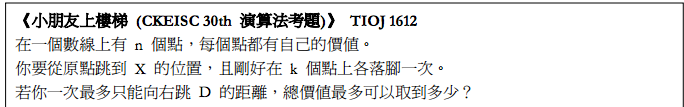# Introduction to deque optimizations

A deque ( double ended queue ) is a data structure that supports push/ pop from both ends with constant time complexity. It can be used to optimize algorithms that deal with monotonous properties. The first time I saw this data structure, I thought it read "de- queue", but later found that it actually is supposed to read "deck", for "dequeue" already has a meaning of "popping out element from queue".
Double-ended queue - Wikipedia, the free encyclopedia

2823 -- Sliding Window
POJ 2823 Sliding Window
Given an array of integers, and a constant k, output minimum and maximum integer in each range that includes exactly k elements.
As the title of the problem suggests, it is a sliding window problem. Let's think about how to maintain the minimum number with deque and the sliding window technique. Scanning from left to right, imagine two integers a and b, a on the left, if a is greater than b, than it will no longer be considered a possible candidate for minimum value on the range, because a will disappear before b will, but before b disappears, a will never have chance to win the minimum. Now we have got the intuition, we will know that we should maintain a deque such that it is strictly increasing monotonically ( strictly but we'll take the latest duplicate ). So that the minimum is always in the front ( leftmost ) in the deque, unless that element is outdated, than it should be popped out, and the opportunity to become the minimum will be handed to the second front.

```#include <cstdio>
#include <algorithm>
#include <deque>
using namespace std;
const int MAXN = 1e6 + 6;

int n, k;
int a[MAXN];

int minv[MAXN], maxv[MAXN];
void solve(){
deque<int> dqmin, dqmax;
for(int i = 0; i < n; ++i){
while( !dqmin.empty() && dqmin.front() < i - k + 1 )
dqmin.pop_front(); // say goodbye to outdated
while( !dqmin.empty() && a[ dqmin.back() ] >= a[i] )
dqmin.pop_back(); // say goodbye to those violating monotony
dqmin.push_back( i );
if( i - k + 1 >= 0 ) // the range is valid
minv[i - k + 1] = a[ dqmin.front() ]; // the front is always the minimum

while( !dqmax.empty() && dqmax.front() < i - k + 1 )
dqmax.pop_front();
while( !dqmax.empty() && a[ dqmax.back() ] <= a[i] )
dqmax.pop_back();
dqmax.push_back( i );
if( i - k + 1 >= 0 )
maxv[i - k + 1] = a[ dqmax.front() ];
}
for(int i = 0; i <= n - k; ++i)
printf("%d%c", minv[i], i == n - k ? '\n' : ' ');
for(int i = 0; i <= n - k; ++i)
printf("%d%c", maxv[i], i == n - k ? '\n' : ' ');
}

int main(){
scanf("%d %d", &n, &k);
for(int i = 0; i < n; ++i)
scanf("%d", &a[i]);
solve();
return 0;
}
```

1612 - Problem F 小朋友上樓梯 (JUMPUP) | TIOJ INFOR Online Judge Found this problem from:
http://pisces.ck.tp.edu.tw/~peng/index.php?action=showfile&file=fcaa846ddcba22c1c63777723152ba9492a9f2218A brief summary for the problem from the site.
DP relation:
maxp[ i ][ j ]: max sum points possible when currently on i'th floor ( x[ i ] ), having stepped j floors already.
maxp[ i ][ j ] = max{ maxp[ k ][ j - 1 ] } + v[ i ], Ɐ k < i && x[ k ] + D ≥ x[ i ] or -INF, if ∄ such k
In the beginning I tried constructing the deque from 0 to n, but failed realizing that the conditions are hard to be maintained that way. However, doing it backwards is easy. We will push things from right to left, until the limit where the conditions satisfy, while popping out things that are obsolete ( ex. when updating some maxp[ i ][ j ], all maxp[ k ][ j - 1 ] where k >= i cannot be used anymore.

```#include <bits/stdc++.h>
using namespace std;
typedef long long ll;
typedef pair<ll, int> pli;
const ll INF = 1LL << 60;
const int MAXN = 3000 + 3;

int n, k;
ll X, D;
pli xv[MAXN];
cin >> n >> k >> X >> D;
for(int i = 0; i < n; ++i)
cin >> xv[i].first >> xv[i].second;
}

ll maxp[MAXN];

void enqueue(deque<int> &deq, int i, int &k){
for( ; k >= 0 && xv[ k ].first + D >= xv[ i ].first; --k){
while( !deq.empty() && maxp[ deq.front() ] <= maxp[ k ] )
deq.pop_front();
deq.push_front( k );
}
}

void dequeue(deque<int> &deq, int i){
while( !deq.empty() && deq.back() >= i )
deq.pop_back(); // outdated
} // updating by descending order => Ɐ k >= i is outdated

void solve(){
xv[ n ] = pli( 0LL, 0 ); // 転移可能な状態なので入れる
sort( xv, xv + n + 1 );

fill( maxp, maxp + MAXN, -INF );
maxp[ 0 ] = 0LL; // 一つ目の転移はこれしかない

for(int j = 0; j < k; ++j){
int cur = n - 1;
deque<int> deq; // これでmaxp[*][j - 1] のmax の単調性をなんとか
for(int i = n; i >= 0; --i){
enqueue( deq, i, cur );
dequeue( deq, i );
if( deq.empty() ) // maxp[i][j - 1] に転移可能な状態がない
maxp[ i ] = -INF;
else // 単調性でback() が常に最大と
maxp[ i ] = maxp[ deq.back() ] + xv[ i ].second;
}
}

int max_idx = -1;
for(int i = n; i >= 0 && xv[ i ].first + D >= X; --i)
if( max_idx < 0 || maxp[ max_idx ] < maxp[ i ] )
max_idx = i;
cout << maxp[ max_idx ] << endl;
}

int main(){
ios::sync_with_stdio(false);